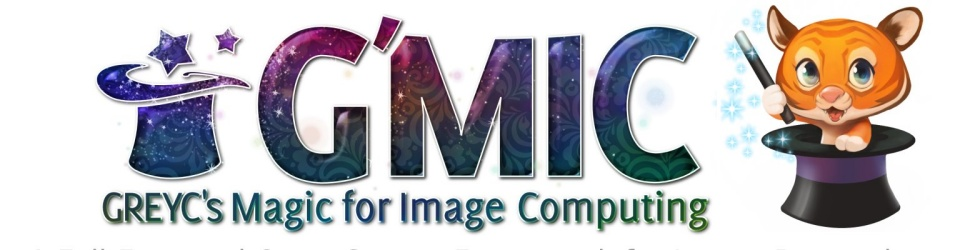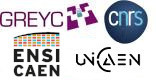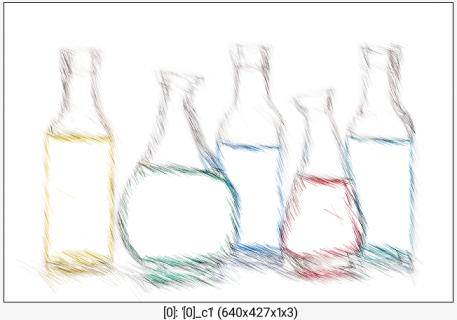G'MIC - GREYC's Magic for Image Computing: A Full-Featured Open-Source Framework for Image ProcessingReference

# sketchbw

## Arguments:

• _nb_angles>0,_start_angle,_angle_range>=0,_length>=0,_threshold>=0,_opacity,_bgfactor>=0,_density>0,_sharpness>=0,_anisotropy>=0,_smoothness>=0,_coherence>=0,_is_boost={ 0 | 1 },_is_curved={ 0 | 1 }

## Description:

Apply sketch effect to selected images.

## Default values:

nb_angles=2, start_angle=45, angle_range=180, length=30, threshold=3, opacity=0.03, bgfactor=0, density=0.6, sharpness=0.1, anisotropy=0.6, smoothness=0.25, coherence=1, is_boost=0 and is_curved=1.

## Example of use:

\$ gmic image.jpg +sketchbw 1 reverse blur[-1] 3 blend[-2,-1] overlayCommand: image.jpg +sketchbw 1 reverse blur[-1] 3 blend[-2,-1] overlay
G'MIC - GREYC's Magic for Image Computing: A Full-Featured Open-Source Framework for Image Processing